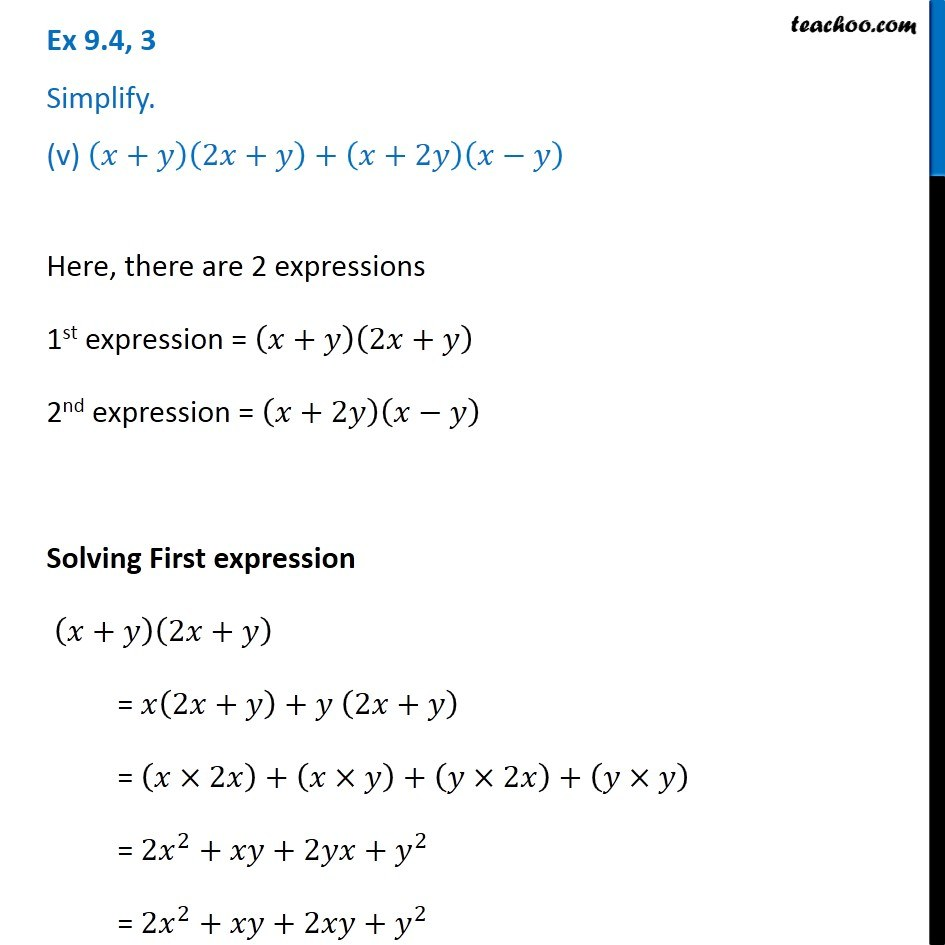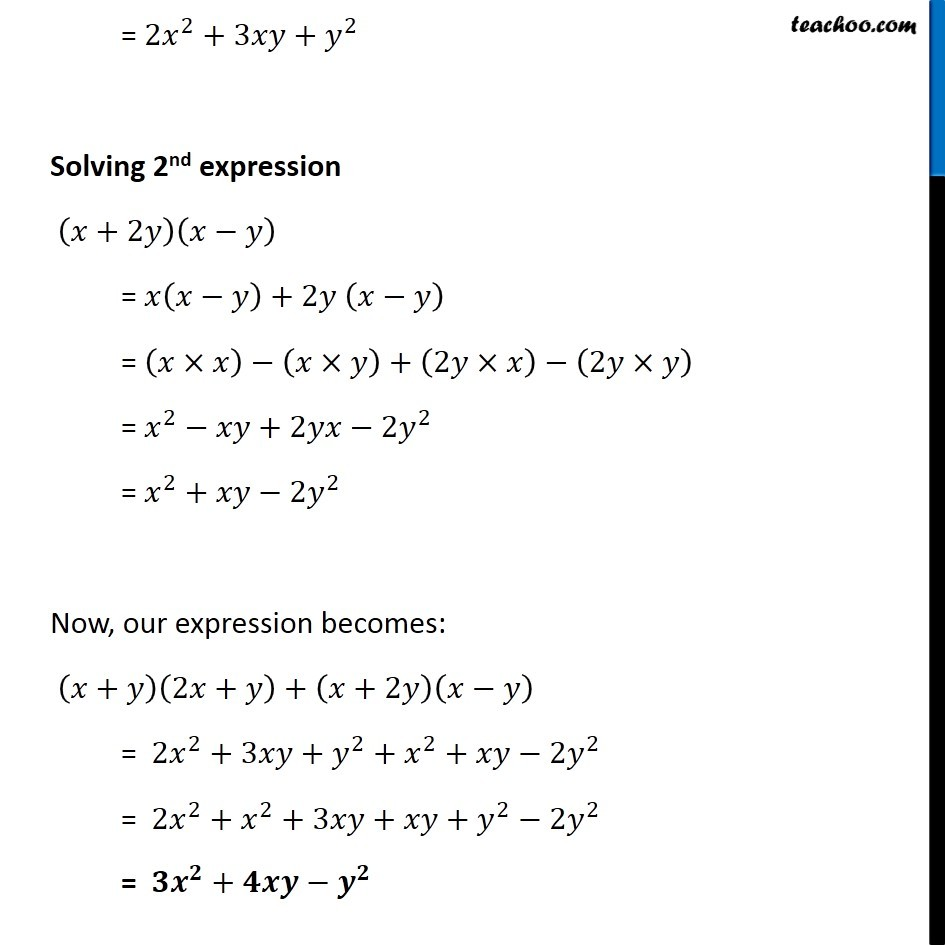Subscribe to our Youtube Channel - https://you.tube/teachoo

1. Chapter 9 Class 8 Algebraic Expressions and Identities
2. Concept wise
3. Multiplication of Polynoimals by Polynomials

Transcript

Ex 9.4, 3 Simplify. (v) (𝑥+𝑦)(2𝑥+𝑦)+(𝑥+2𝑦)(𝑥−𝑦) Here, there are 2 expressions 1st expression = (𝑥+𝑦)(2𝑥+𝑦) 2nd expression = (𝑥+2𝑦)(𝑥−𝑦) Solving First expression (𝑥+𝑦)(2𝑥+𝑦) = 𝑥(2𝑥+𝑦)+𝑦 (2𝑥+𝑦) = (𝑥×2𝑥)+(𝑥×𝑦)+(𝑦×2𝑥)+(𝑦×𝑦) = 2𝑥^2+𝑥𝑦+2𝑦𝑥+𝑦^2 = 2𝑥^2+𝑥𝑦+2𝑥𝑦+𝑦^2 = 2𝑥^2+3𝑥𝑦+𝑦^2 Solving 2nd expression (𝑥+2𝑦)(𝑥−𝑦) = 𝑥(𝑥−𝑦)+2𝑦 (𝑥−𝑦) = (𝑥×𝑥)−(𝑥×𝑦)+(2𝑦×𝑥)−(2𝑦×𝑦) = 𝑥^2−𝑥𝑦+2𝑦𝑥−2𝑦^2 = 𝑥^2+𝑥𝑦−2𝑦^2 Now, our expression becomes: (𝑥+𝑦)(2𝑥+𝑦)+(𝑥+2𝑦)(𝑥−𝑦) = 2𝑥^2+3𝑥𝑦+𝑦^2+𝑥^2+𝑥𝑦−2𝑦^2 = 2𝑥^2+𝑥^2+3𝑥𝑦+𝑥𝑦+𝑦^2−2𝑦^2 = 𝟑𝒙^𝟐+𝟒𝒙𝒚−𝒚^𝟐

Multiplication of Polynoimals by Polynomials